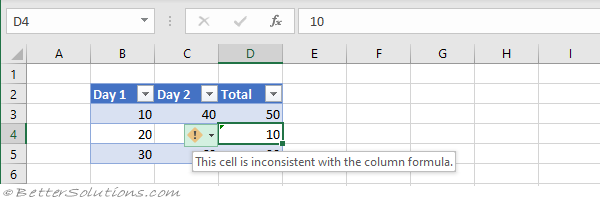# Inconsistent Calculated Column Formula

This rule will identify any cells in a calculated table column that are different to the others in that column.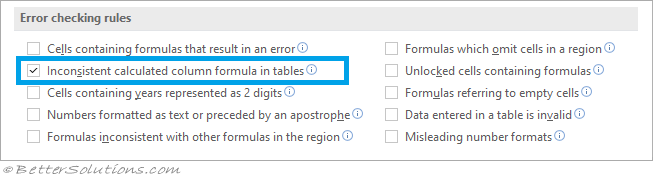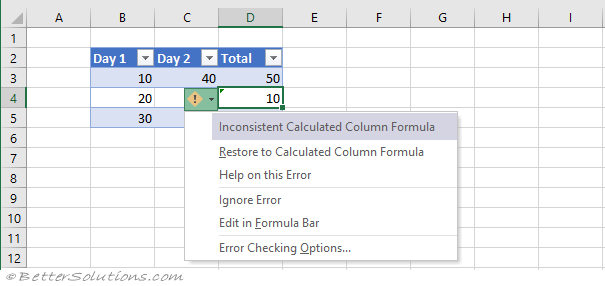Restore to Calculated Column Formula - Updates the formula so that it's consistent with the rest of the column.
Help on this Error - Displays the Help task pane.
Ignore Error - This removes the green triangle from the cell and the cell is no longer considered an error.
Edit in Formula Bar - Places the cursor into the Formula bar so it can be edited.
Error Checking Options - Displays the Options, Formulas Tab.

### Example

When a formula in a calculated table column is different to the others in that column.
Enter the following data into cells "B2:C5".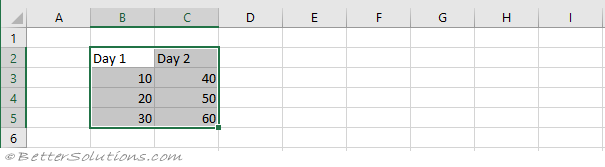Convert this range to a table using the Table command on the Insert Tab.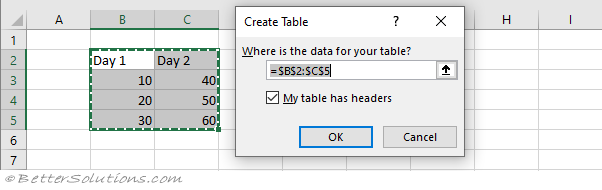Enter the text "Total" into cell "D2".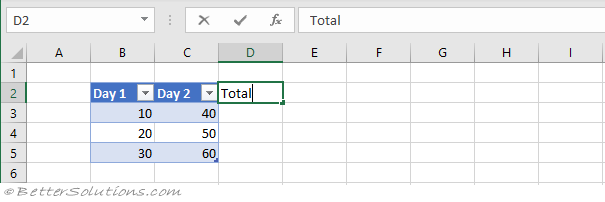The table will be automatically expanded to include this extra column.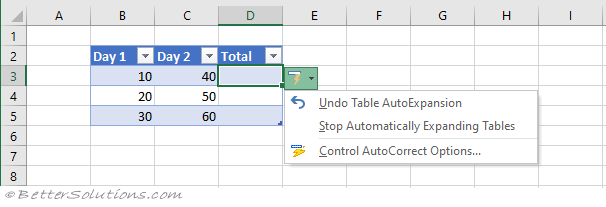Enter the formula "=B3+C3" into cell "D3".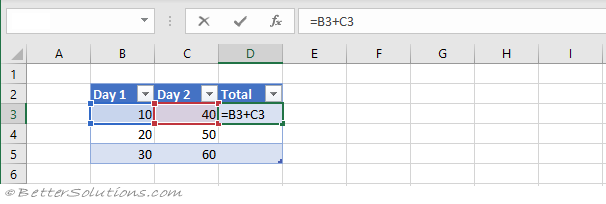The formula will be automatically expanded to the rest of the column.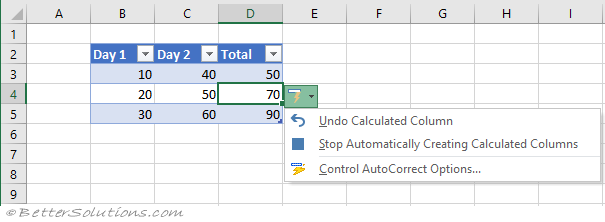Enter the number 10 into cell "D4".